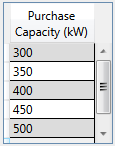# HOMER Pro 3.9Type: Input Variable Units: kW Symbol: Tgrid

The purchase capacity is the maximum amount of power that can be drawn from the grid at any time. It is a decision variable because of the effect of demand charges. HOMER does not explicitly consider the demand rate in its time-step-by-time-step decisions as to how to control the power system; the demand charge is simply calculated at the end of each annual simulation. As a result, HOMER will not turn on a generator simply to save demand charges. But it will turn on a generator whenever the load exceeds the maximum grid demand.

If the demand rate is zero, you need only specify a single value for the maximum grid demand. Normally, the true capacity of the grid is much higher than the system peak load, and the maximum grid demand can be set to any value higher than the system peak load. In the rare case that the grid is unable to meet the system peak load, the maximum grid demand should be set to the actual capacity of the grid.

If the demand rate is not zero, specify a value equal to or greater than the peak load, plus at least one value smaller than the peak load. HOMER will find the optimal value.

The maximum grid demand appears in a table on the Grid window: